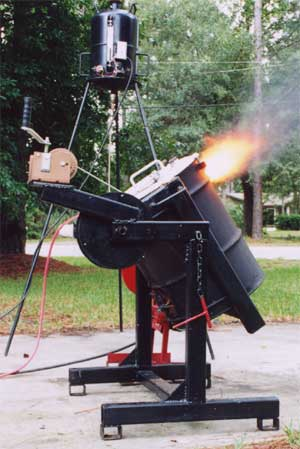# How to Build an Oil Fired Tilting Furnace### 4 TYPES AND EFFCIENCIES OF TYPICAL FURNACES:

Fuel fired furnaces are either direct-fired where the products of combustion come in direct contact with the metal charge as in the case of a reverberatory furnace or indirect-fired where the products of combustion do not come directly in contact with the metal charge as in a crucible furnace. The different types of furnaces influence the melting loss and chemical composition of the aluminum.

The direct-fired reverberatory furnace is an efficient, fast melting, high volume unit. Because of the direct contact with the flame, the reverberatory furnace produces more dross and has a higher rate of gas absorption. Large reverberatory furnaces are claimed to melt with as little as 1500 Btu per pound and have a melting capacity of 40 pounds of aluminum per square foot of bath area per hour.

Crucible furnaces are not as efficient as reverberatory furnaces because the heat must be transferred through the walls of a crucible to the aluminum. Crucible furnaces come in three types, the lift out crucible type, where the crucible is used as a ladle, the stationary crucible type where the aluminum is dipped out with a ladle and the tilting type where the metal is poured directly from the furnace.

Crucible furnaces generally have between 3 to 5 inches of refractory that is rammed or poured inside a steel shell.

The efficiency of crucible furnaces range from a low 3.5% to a high of 28% the common commercial average being around 15%. The average heat input to melt aluminum is approximately 3300 Btu per pound.

EFFICIENCY OF FURNACES: The efficiency of a furnace is the ratio of heat input to useful output. To calculate the furnace efficiency you divide the theoretical amount of heat needed by the actual amount of heat used to melt a specific amount of metal.

Efficiency = Theoretical Btu / Actual Btu

Calculate the efficiency of an oil-burning furnace that uses 2 gallons of fuel oil rated at 130,600 Btu per gallon to melt 50 pounds of aluminum to a pouring temperature of 1380deg. F:

From pages 16 & 17, the theoretical Btu content of aluminum at 1380 deg. F = 498 Btu / pound

Total Btu content of the metal = Weight x Btu / pound

Total Btu = 50 pounds x 498 Btu / pound = 24,900 Btu

The total number of Btu consumed in the furnace is calculated by multiplying the number of gallons times the Btu content per gallon.

Btu = Gallons x Btu / gallon

Btu = 2 gallons x 130,600 Btu / gallon = 261,200 Btu

Now to find the efficiency of the furnace:

Efficiency = 100 x (Theoretical Btu / Actual Btu)

Efficiency = 100 x (24,900 Btu / 261,200 Btu) = 9.53%

The furnace efficiency is 9.53%

This calculation may be repeated for any type of fuel, furnace or metal by substituting the proper values for the fuel and metal.

Read More on Melting Practice

Author: Steve Chastain

This div height required for enabling the sticky sidebar